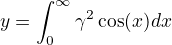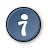AsciiDoc
Text based document generation

The AsciiDoc distribution includes a LaTeX filter that translates LaTeX source to an image which is automatically inserted into the AsciiDoc output document. Although it can accept any LaTeX source, the primary use is to render mathematical formulae (see the examples below). The filter implements the latex Listing block and Paragraph styles.

Two image formats are supported; PNG and SVG. PNG is the default since that was the first format that this filter supported. However, SVG is a better format since it’s scalable. Using SVG make formulas look good in both PDFs and on web pages. SVG will also scale well when zooming in on a web page for example. It is recommended to always use the SVG format. This can be done by setting the imgfmt parameter to svg, as is done below. An even better way is to set the global attribute latex-imgfmt to svg. Then SVG will be used for all formulas.

This LaTeX paragraph:

["latex", imgfmt="svg"]
---------------------------------------------------------------------
\begin{equation*}
y = \int_0^\infty \gamma^2 \cos(x) dx
\end{equation*}
---------------------------------------------------------------------

Renders:Compare the formula above, which is rendered as an SVG image, to the formula below which has been rendered as a PNG image. The difference will be most notable if zooming in on a web page, printing the web page or when rendering the document as a PDF.

["latex", "latex2.png", 140, imgfmt="png"]
---------------------------------------------------------------------
\begin{equation*}
y = \int_0^\infty \gamma^2 \cos(x) dx
\end{equation*}
---------------------------------------------------------------------

Renders:This LaTeX block:

["latex","latex1.svg",imgfmt="svg",align="center"]
---------------------------------------------------------------------
\begin{equation*}
\displaystyle{ V_i = C_0 - C_3
\frac{C_1\cos(\theta_i+C_3)}{C_4+C_1\cos(\theta_i+C_2)} }
\end{equation*}
---------------------------------------------------------------------

Renders:This LaTeX block:

.LaTeX filter example
[latex]
["latex","latex3.svg",imgfmt="svg"]
---------------------------------------------------------------------
\begin{equation}
\Re{z} =\frac{n\pi \dfrac{\theta +\psi}{2}}{
\left(\dfrac{\theta +\psi}{2}\right)^2 + \left( \dfrac{1}{2}
\log \left\lvert\dfrac{B}{A}\right\rvert\right)^2}.
\end{equation}

\begin{equation}
\boxed{\eta \leq C(\delta(\eta) +\Lambda_M(0,\delta))}
\end{equation}

\begin{equation}\label{first}
a=b+c
\end{equation}

\begin{subequations}\label{grp}
\begin{align}
a&=b+c\label{second}\\
d&=e+f+g\label{third}\\
h&=i+j\label{fourth}
\end{align}
\end{subequations}
---------------------------------------------------------------------

Renders:Figure 1. LaTeX filter example

This LaTeX paragraph:

.A LaTeX table
["latex",imgfmt="svg"]
\begin{tabular}{c r @{.} l}
Pi expression &
\multicolumn{2}{c}{Value} \\
\hline
$\pi$ & 3&1416 \\
$\pi^{\pi}$ & 36&46 \\
$(\pi^{\pi})^{\pi}$ & 80662&7 \\
\end{tabular}

Renders:Figure 2. A LaTeX table

## Using the Filter

• The LaTeX filter is invoked by setting the Listing block or Paragraph style (the first positional block attribute) to latex.

• The second positional attribute (named target is optional, it sets the name of the generated image file. If this is not supplied a file name like {docname}__{target-number}.{imgfmt} is synthesised (where {docname} is the document file name, {target-number} is an integer number and {imgfmt} is the image format (png or svg).

• The third positional attribute, named dpi, is also optional; it is an integer number that sets the output resolution in dots per inch for a PNG image. It is ignored for an SVG image.

• The image format to use can be selected by setting the imgfmt parameter or by globally setting the latex-imgfmt attribute. Setting it to svg will render SVG images and setting it to png will render PNG images. The default is png.

Because the LaTeX images are rendered using the image block templates you can also use the optional named image block attributes (see Image macro attributes in the AsciiDoc User Guide).You can also change the image size using the following LaTeX commands: \tiny \scriptsize \footnotesize \small \normalsize \large \Large \LARGE \huge For example: [latex] \Large $y = \int_0^\infty \gamma^2 \cos(x) dx$ The \Large command is outside the $ math delimiters. The filter (./filters/latex/latex2img.py) can be used outside AsciiDoc to convert LaTeX source to images. Execute the following command to see how to use it: $ ./filters/latex/latex2img.py --help

## Limitations

• The asciidoc(1) input and output files cannot both be - (stdin and stdout), either the input or output files (or both) must be a named file.

## Installation

In addition to AsciiDoc you will need to have latex(1), dvipng(1) (for PNG) and/or dvisvgm(1) (for SVG) installed.